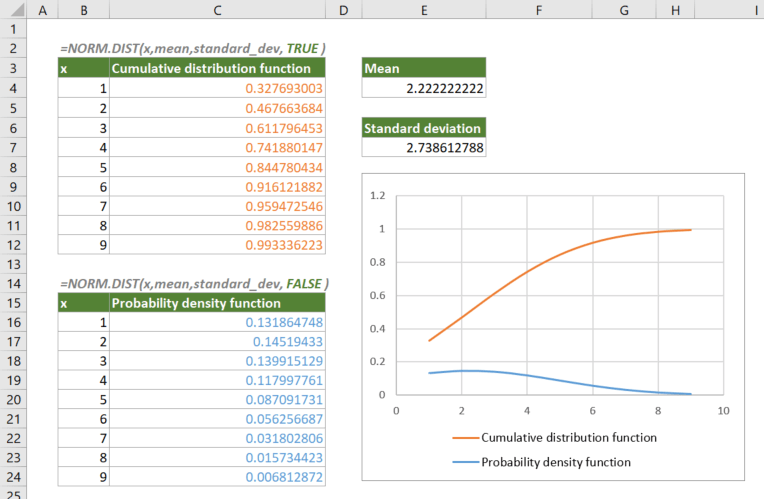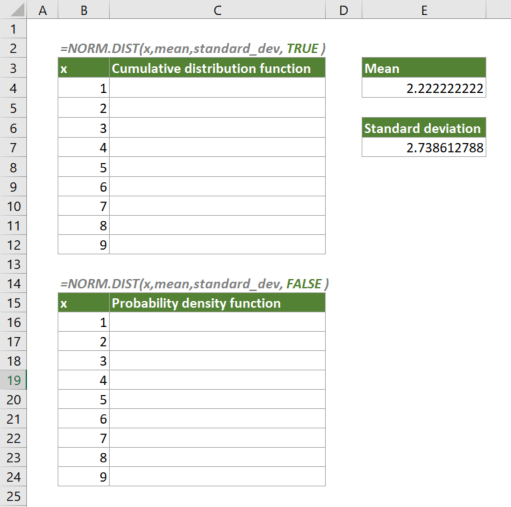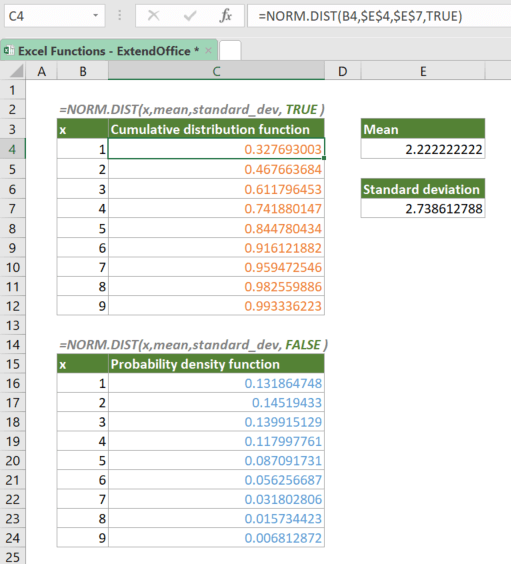## Excel NORM.DIST Function

The NORM.DIST calculates and returns the standard normal cumulative distribution function or probability density function of a value for the given arithmetic mean and standard deviation.#### Syntax

=NORM.DIST(x, mean, standard_dev, cumulative)

#### Arguments

• x (required): The value for which to calculate the distribution.
• mean (required): The arithmetic mean of the distribution.
• standard_dev (required): The standard deviation of the distribution.
• cumulative (required): A logical value that specifies the type of distribution to be used:
• TRUE: Returns the cumulative distribution function (CDF);
• FALSE: Returns the probability density function (PDF).

#### Return Value

The NORM.DIST function returns a numeric value.

#### Function Notes

• The #VALUE! error occurs if x, mean or standard_dev is nonnumeric.
• The #NUM! error occurs if standard_dev ≤ 0.

#### ExampleSupposing you have a list of values (x) as shown above, their mean and standard deviation are calculated and returned at the right side of the table. To get the cumulative distribution function of the same set of values based on the mean and standard deviation, please copy or enter the formula below in cell C4, and press Enter to get the result:

=NORM.DIST(B4,\$E\$4,\$E\$7,TRUE)

√ Note: The dollar signs (\$) above indicate absolute references, which means the array argument in the formula won't change when you move or copy the formula to other cells. However, there are no dollar signs added to x since you want it to be dynamic. After entering the formula, drag the fill handle down to apply the formula to the below cells.

To get the probability density function, please copy or enter the formula below in cell C16, press Enter to get the result, and then drag the fill handle down to apply the formula to the below cells:

=NORM.DIST(B16,\$E\$4,\$E\$7,FALSE)#### NORM.DIST VS. NORM.S.DIST

• NORM.DIST uses the normal distribution with the mean and standard deviation you specified;
• NORM.S.DIST uses the standard normal distribution which is a special case of the normal distribution where the mean is 0 and the standard deviation is 1.

#### Related functions

Excel NORM.INV Function

The NORM.INV calculates and returns the inverse of the normal cumulative distribution for the given arithmetic mean and standard deviation.

Excel NORM.S.DIST Function

The NORM.S.DIST calculates and returns the standard normal cumulative distribution function or probability density function of a value for an arithmetic mean of 0 and standard deviation of 1.

Excel NORM.S.INV Function

The NORM.S.INV calculates and returns the inverse of the standard normal cumulative distribution that has an arithmetic mean of 0 and standard deviation of 1 with a given probability.

### The Best Office Productivity Tools

#### Kutools for Excel - Helps You To Stand Out From Crowd

 Popular Features: Find/Highlight/Identify Duplicates  |  Delete Blank Rows  |  Combine Columns or Cells without Losing Data  |  Round without Formula ... Super VLookup: Multiple Criteria  |  Multiple Value   |  Across Multi-Sheets  |  Fuzzy Lookup... Adv. Drop-down List: Easy Drop Down List  |  Dependent Drop Down List  |  Multi-select Drop Down List... Column Manager: Add a Specific Number of Columns  |  Move Columns  |  Unhide Columns  |  Compare Columns to Select Same & Different Cells ... Featured Features: Grid Focus  |  Design View  |  Big Formula Bar  |  Workbook & Sheet Manager  |  Resource Library (Auto Text)  |  Date Picker  |  Combine Worksheets  |  Encrypt/Decrypt Cells  |  Send Emails by List  |  Super Filter  |  Special Filter (filter bold/italic/strikethrough...) ... Top 15 Toolset:  12 Text Tools (Add Text, Remove Characters ...)  |  50+ Chart Types (Gantt Chart ...)  |  40+ Practical Formulas (Calculate age based on birthday ...)  |  19 Insertion Tools (Insert QR Code, Insert Picture from Path ...)  |  12 Conversion Tools (Numbers to Words, Currency Conversion ...)  |  7 Merge & Split Tools (Advanced Combine Rows, Split Cells ...)  |  Many More...

Kutools for Excel Boasts Over 300 Features, Ensuring That What You Need is Just A Click Away...

Supports Office/Excel 2007-2021 and 365   |   Available in 44 Languages   |   Full-Featured 30-Day Free Trial.#### Office Tab - Enable Tabbed Reading and Editing in Microsoft Office (include Excel)

• One second to switch between dozens of open documents!
• Reduce hundreds of mouse clicks for you every day, say goodbye to mouse hand.
• Increases your productivity by 50% when viewing and editing multiple documents.
• Brings Efficient Tabs to Office (include Excel), Just Like Chrome, Firefox, And New Internet Explorer.